# Module 1: INITIAL SETUP AND BASIC OPERATION

## Learning Objectives

After reading this lesson, you should be able to:

1. know the main components of the MATLAB environment,

2. use the MATLAB software to write code,

3. use the interface to find and set the current working location of MATLAB.

## MATLAB Environment Windows and Parts

The MATLAB Environment is all the windows, sub-windows, and parts that are part of the MATLAB program (note, both the software interface and the actual computer language are called MATLAB). By default, the components of the MATLAB Environment are laid out as shown in Figure 1. Note that we are referencing MATLAB versions R2015a and above in this lesson. Previous versions have some similarities, but R2012a and before are significantly different visually (no ribbon navigation). However, the basic parts like the Editor and Command Window are fundamentally the same throughout the different versions.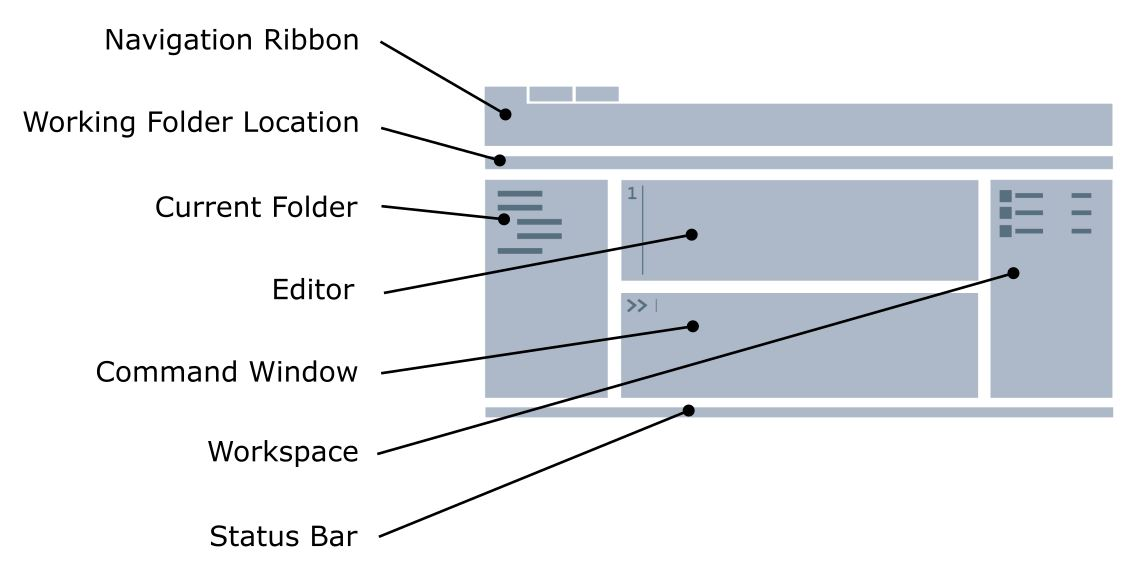Figure 1: The major windows and section of the default setup of the MATLAB Environment.

The following sections of this lesson will review the individual and major parts of the MATLAB Environment seen above in Figure 1. You can refer back to Figure 1 and/or open MATLAB to see physically where the different pieces are as you progress through this lesson. It is essential for a beginner to understand each component and its function to write MATLAB code.

## Working Folder Location

The working folder location bar shows the current directory that MATLAB is referencing (see Figure 3). This is the computer folder from which your code will run. If the working directory is different than the location of your code, MATLAB will prompt to change the working folder location (shown in Figure 4). The working folder location bar works in conjunction with the current folder window, which is explained in the following section.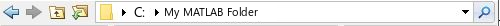Figure 3: The working folder display in MATLAB.

Figure 4 shows the prompt you will get if the m-file you are trying to run is not in the MATLAB current folder or on one of its (directory) paths. “Change Folder” means: make the folder that contains this m-file the “current folder”. “Add to Path” means add the folder location of the m-file you are trying to run to the MATLAB paths. A path is a more permanent directory that MATLAB will always look in when trying to find a program or function you are running/calling. Simply put, choose “Change Folder” most of the time/if you do not know. Neither option will “break” anything, and both should work fine for our purposes.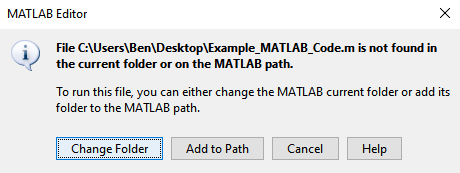Figure 4: MATLAB user prompt to change the current working folder.

## Current Folder

The current folder MATLAB Environment sub-window, shown by default on the right-hand side of the window, shows you what files are in your working folder location (see Figure 5). This includes all subfolders and any type of files that are inside the working directory.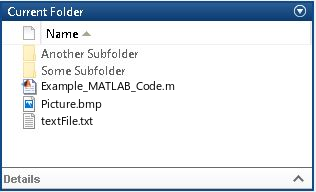Figure 5: Current folder contents window in MATLAB.

## Command Window

The Command Window has a number of different uses in MATLAB. Generally, it acts as an output window and a temporary/quick coding environment. We will cover this window in depth in Lesson 1.6.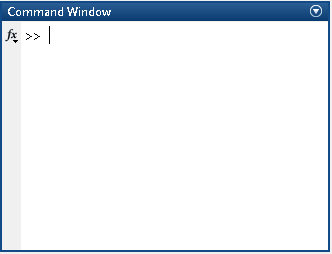Figure 6: MATLAB Command Window.

## Editor Window

While the Command Window can act as a temporary coding environment, the MATLAB Editor window is used to write outlines of code, or a script, that can be executed all at the same time. You can write an entire code sequence before running it. We will cover this window more in-depth in the following lesson on the m-file (Lesson 1.5). This window should not be confused with the Editor tab. They are related, but not the same thing as you can see in MATLAB.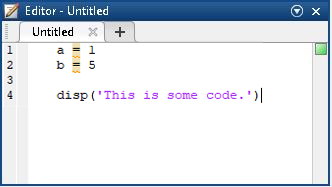Figure 7: The Editor window (m-file editor) used to write and save a complete code.

## Workspace

While programming, you will actively define variables to store data and values for later use. Such memory storage can range from storing text and numbers to storing large vectors and matrices. The workspace window (Figure 8) displays all variables that are currently defined and stored into memory. It can be helpful in that it shows different properties of that particular variable such as its type (“Class”), its matrix dimensions (“Size”), or the amount of memory used to store it (“Bytes”). You can even see quick facts about numerical data like the mean of the data.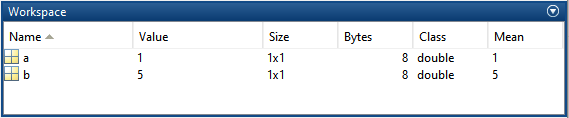Figure 8: The workspace window that is used to monitor any MATLAB data currently loaded into memory.

## Status Bar

The status bar indicates the active status of the MATLAB program. Most of the time, there will be nothing important to display here. However, one particularly useful message is when MATLAB tells you it is “Busy” or “Waiting for an input”. “Busy” generally means it is working on computations in the background from the last code you asked it to run. “Waiting for an input” refers to MATLAB waiting for a user input that you specified in your program (more on this in Lesson 2.4: Inputs and Outputs).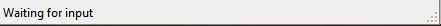Figure 9: The status bar that notifies the user of important MATLAB status messages.